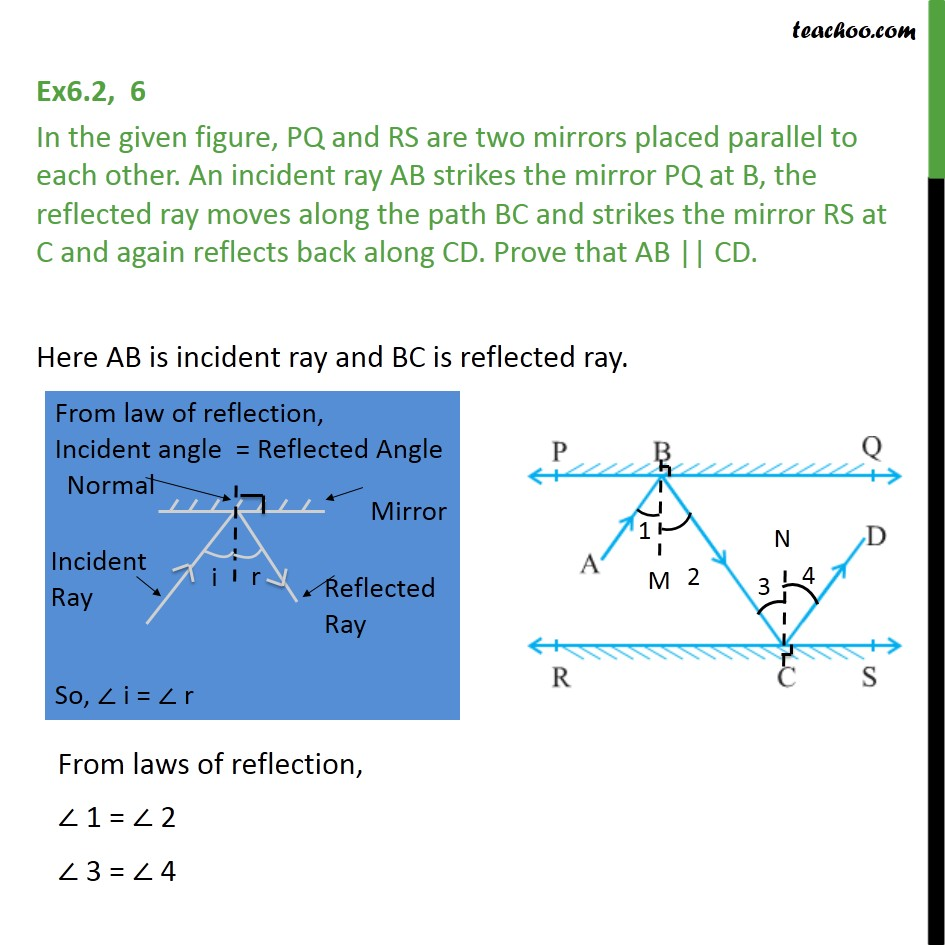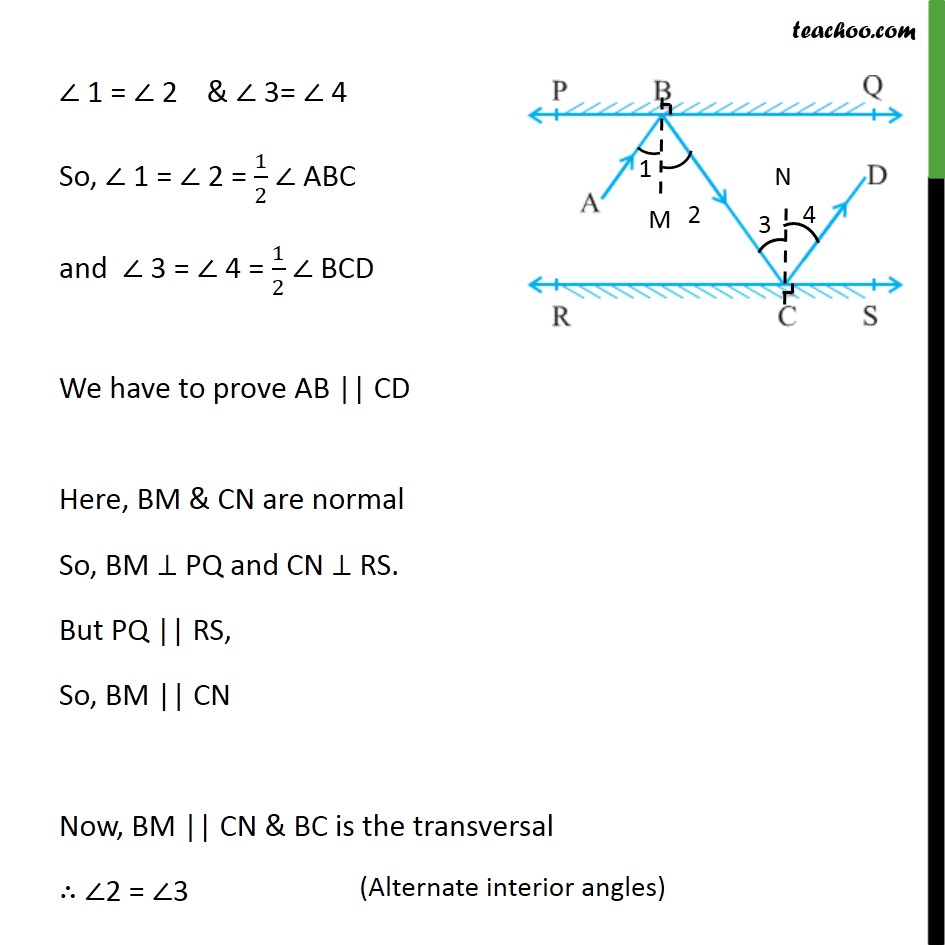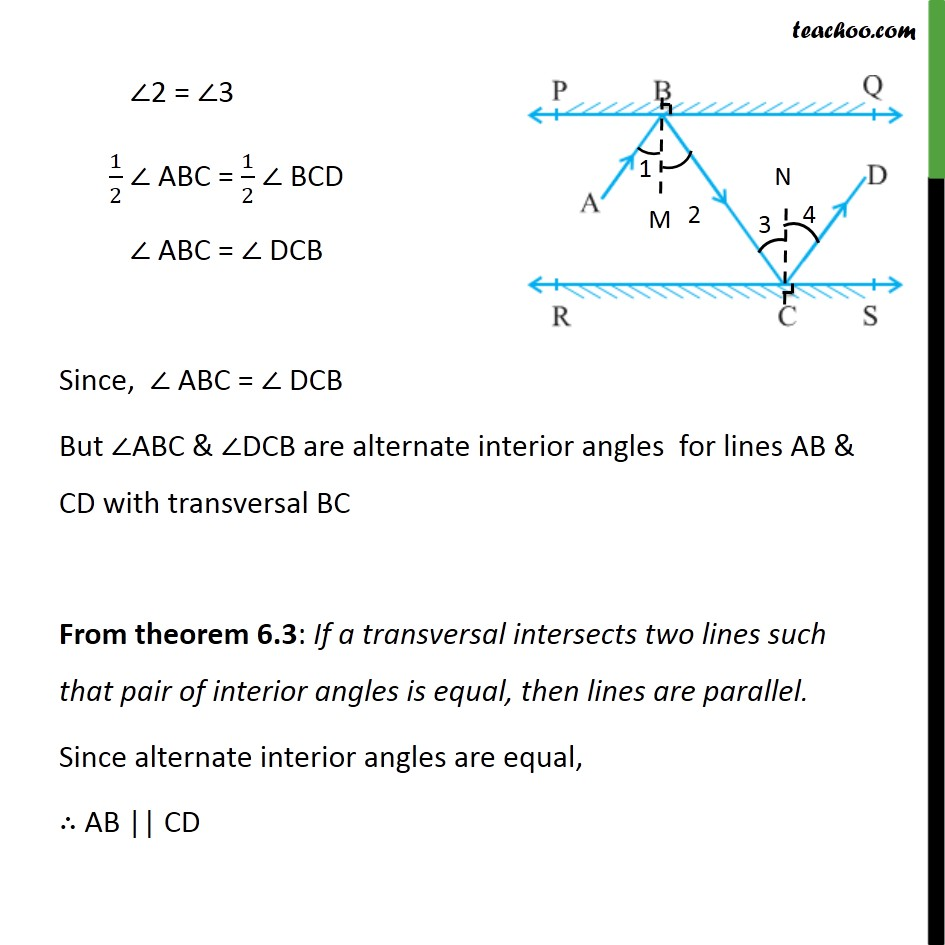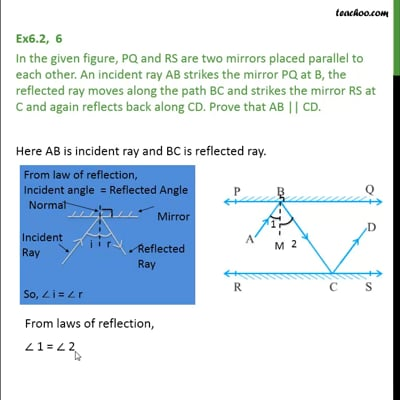Ex 6.2

Chapter 6 Class 9 Lines and Angles
Serial order wiseThis video is only available for Teachoo black users

Maths Crash Course - Live lectures + all videos + Real time Doubt solving!

### Transcript

Ex6.2, 6 In the given figure, PQ and RS are two mirrors placed parallel to each other. An incident ray AB strikes the mirror PQ at B, the reflected ray moves along the path BC and strikes the mirror RS at C and again reflects back along CD. Prove that AB || CD. Here AB is incident ray and BC is reflected ray. From laws of reflection, 1 = 2 3 = 4 1 = 2 & 3= 4 So, 1 = 2 = 1/2 ABC and 3 = 4 = 1/2 BCD We have to prove AB || CD Here, BM & CN are normal So, BM PQ and CN RS. But PQ || RS, So, BM || CN Now, BM || CN & BC is the transversal 2 = 3 2 = 3 1/2 ABC = 1/2 BCD ABC = DCB Since, ABC = DCB But ABC & DCB are alternate interior angles for lines AB & CD with transversal BC From theorem 6.3: If a transversal intersects two lines such that pair of interior angles is equal, then lines are parallel. Since alternate interior angles are equal, AB || CD